Courses

# Coding - Decoding LR Notes | EduRev

## CLAT : Coding - Decoding LR Notes | EduRev

The document Coding - Decoding LR Notes | EduRev is a part of the CLAT Course General Intelligence and Reasoning for SSC CHSL.
All you need of CLAT at this link: CLAT

A CODE is a signal. Coding has always been used to transmit messages in such a way that they are not ordinarily understood, unless these are decoded. These messages can only be understood by the receiver if he has the ‘code key’ to decipher it. Thus, the “CODING DECODING” test is given to judge the candidate’s ability to decipher the ‘rule applied in coding’.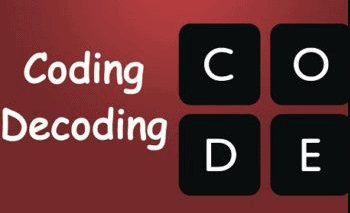The coding is generally based on alphabets and some times numerals are also used to code the messages.
Though, all the methods of coding cannot be identified but still the major formats of coding are as under:

Type 1: Coding based on alphabet.
Type 2: Coding based on symbols and numbers.
Type 3: Coding based on renaming.
Type 4: Coding based on group of words.
Type 5: Miscellaneous.

TYPE – 1: CODING BASED ON ALPHABET
(A) Reversal Of Alphabets
The alphabets of the word are reversed in such a way that the last letter is the code of first letter. It is the easy format of the coding and it is the first thing to be looked when we look at coding.

Example 1: In a certain code language BREAKDOWN is written as NWODKAERB. How will TRIANGLES be written in that code language?
(1) AIRTGNSEL
(2) SELGWTRIA
(3) AIRTNSELG
(4) SELGNAIRT
Sol. (4)
The order of letters has been reversed in the code.
Following the coding given above, TRIANGLES will be Coded as SELGNAIRT.

Example 2: In a certain code ORDER is written as REDRO. Which of the following represents SUBSTITUTION in that code?
(1) NOITUTITSBUS
(2) TITUTIONSBUS
(3) NOITTITUSBUS
(4) NOITSBUSTITU
Sol. (1)
The order of letters has been reversed in the code:
Following the coding given above SUBSTITUTION will be coded as NOITUTITSBUS.

(B) Opposite Letters
The second type of coding is by ‘use of opposite letters’. We can understand this type from the rule of 27, which informs us how to find out the opposite letters of the alphabet. Knowing the position value of each alphabet and using the second rule of 27, we can easily find out if the word is coded in ‘opposite letters’. Here A will be coded as Z. Therefore, B will be coded as Y and C will be coded as X.

Example 1: In a certain code ORDER is written as LIWVI. How is SUBSTITUTION written in that code?
(1) HFYHGRGFGRLM
(2) HFHYGRGRLM
(3) HFYHGRGRML
(4) HFYHGRHGGQLM
Sol: (1)
A perusal of the word ORDER and its coded word LIWVI and knowledge of second rule of 27 shows that L is opposite letter of O, I is opposite of R, W is opposite of R and so on. It means that the coded word contains all the opposite letters. Keeping this in view, we know that SUBSTITUTION will be coded as HFYHGRGFGRLM.

Example 2: In a certain code COMBINE is written as XLNYRMV. Which of the following represents FLOWER in that code?
(1) UOLDVI
(2) UOLENI
(3) UOKDNH
(4) UOKDNI
Sol. (1)
A perusal of the word COMBINE and its coded word XLNYRMV combined with knowledge of second rule of 27, we know that X is opposite letter of C, L is opposite of O, N is opposite of M and so on. It shows that the coded word contains all the opposite letters. Keeping this in view, we know that FLOWER will be coded as UOLDNI.

(C) Preceding / Following
In this type of questions either preceding or following letter stands as the code letter for each of the letters of the given word. For example,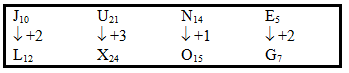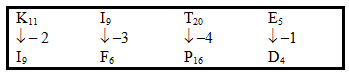Example 1: In a certain code language, ‘TEMPORARY’ is written as ‘SFLQOQBQZ. How would ‘WONDERFUL’ be written in the same code?
(1) VPMEFQGTM
(2) VPMEESGTM
(3) XPMEEQGTM
(4)  None of these
Sol. (4)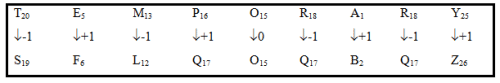Similarly,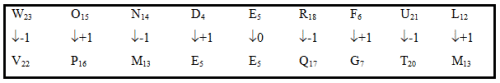We observe that letters are alternatively moved one place forward and one place backwards and the letter in the middle of the given word is not changed. Based on this observation we coded the word ‘WONDERFUL’ as ‘VPMEEQGTM’. Therefore the ans. is (4).

Example 2: In a certain code language DISTANCE is written as ‘FLUWCQEH’. How will ‘CHILDREN’ be written in that code?
(1) EKKOFUGQ
(2) EJKOFUGQ
(3) EKLOFUGQ
(4) EJKNFUGQ
Sol. (1)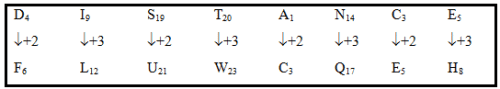Similarly,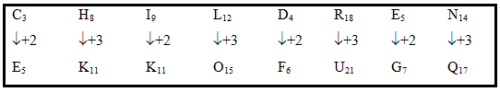If we compare the place value of the coded word with its code we observe that letters are alternatively moved two places and three places forward as shown below. Based on this observation the word ‘CHILDREN’ will be coded as EKKOFUGQ.

(D) Jumbling Of Letters
In this type of coding, the letters of the given word are moved in a particular way and for the purpose of decoding, the same format is required to be followed. In jumbling also a series has to be there.

Example 1: In a certain code language AUTHORITY is written as YTUROHTIA. How will DESIGNATE be written in that code language?
(1) ESENGATDI
(4) ESNEIGTDA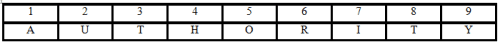Sol. (3)
In the coded form the first and the last (ninth) letters have been interchanged so as the second and third, the fourth and the sixth and the seventh and the eight letters while middle letters (i.e. fifth) is left intact. Thus,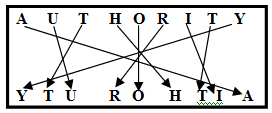Similarly,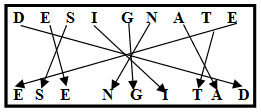Example 2: In a certain code EXPLAIN is written as NPXLIAE. How is PERTAIN written in that code?
(1) NRETIAP
(2) PRETIAN
(3) NERTIAP
(4) NRETAIP
Sol. (3)
We observe that the letters in the word EXPLAIN have been jumbled as under: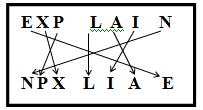Similarly,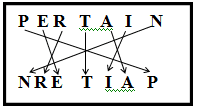Example 3: If ALIMONY is written as YILMNOA then how DISGUISE is written as
(1) ESIUGSID
(2) ESUIGSID
(3) ESIUGSDI
(4) SEUISDID
Sol. (1)
We observed that the letters in the word ALIMONY have been jumbled as under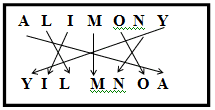Similarly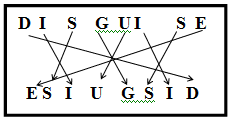Example 4: If HEALING is written as BFIKHOJ; how is BEDTIME written in that code?
(1) EFCSTNF
(2) EFCSFNJ
(3) EFCOFNS
(4) CFESFNJ
Sol. (2)
We observed that the letters in the word HEALING have been jumbled as under: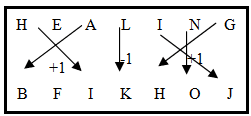Similarly,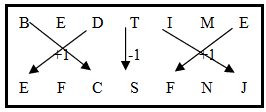Example 5: If MARXIST in written as ARMT (SIX), then how SUNNETS in written in that code?
(1) ONSS (NET)
(2) NOSS (TEN)
(3) NOSS
(4) ONSS (TEN)

Sol. (4)
We observed that the letters in the word MARXIST have been jumbled as under: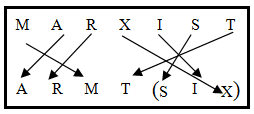Similarly,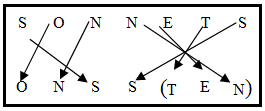It is some time observed that the given word is coded in numeric form. Generally the place values of the letters used in the word are added up and the resultant sum is the coded form of the given word. If the word CAT is coded as 24 because the place value of all the letters are added up.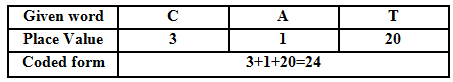Example 1: If MADRAS is coded as 56, how is CALCUTTA coded in that language?
(1) 80
(2) 81
(3) 82
(4) 76
Sol. (2)
The place value of all the letters is added up.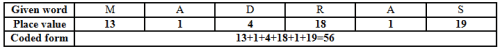Similarly Calcutta can be coded in the same way as: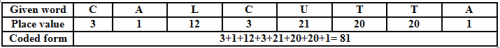Example 2: In a certain code SUMAN is written as 68. How will you write NISHI in that code?
(1) 59
(2) 60
(3) 58
(4) 56

Sol. (1)
We observed that place values of all the letters in the given word SUMAN are added up.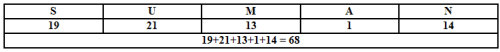Similarly, we will add the place value of all the letters used in the word NISHI and the answer is 59.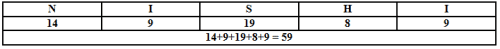Example 3: In a certain code ABDUCT is written as 51. How is FLAKE written in that code?
(1) 36
(2) 35
(3) 34
(4) 33

Sol. (2)
We are given that the word is coded in the format where the place value of all the letters used in word are added up. Similarly we will add the place value of the letters used in the word FLAKE and the answer is 35.

(F) Given Two Words & To Find Code For Third

Example 1: If the word ‘NUMBER’ is coded as ‘EPRSAY’ and ‘SQUARE’ is coded as ‘NGPVYA’ then how do we code ‘MEMBER’.
(a) RARSYA
(b) RRASYA
(c) RARSAY
(d) SARSAY
Sol. (3)
When we observe the coded word and the corresponding, code it is observed that the coding is neither in the form of opposite letters; nor correspond place value in the alphabet. The only thing common between the two coded words are the letters U and E and these letters are coded as P and A respectively in both the given words. Their placement in the code corresponds to their position in the coded words.

Based on this information, we draw the table as shown below and find the code for each letter: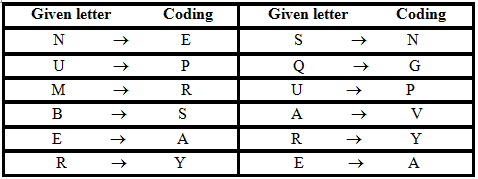If we observe from the table above table, M is coded as R, E is coded as A, B is coded as S. So the answer is RARSAY.

Example 2: Based on the information given above, how the word REAR is coded?
(1) YAXV
(2) XYAV
(3) AVXY
(4) YAVY
Sol. (4) If we observe the above table, R is coded as Y, E is coded as A, A is coded as V. So the answer is YAVY.

TYPE – 2: CODING BASED ON SYMBOLS AND NUMBERS

In the symbol coding, we use the symbols like “!@#\$%^&*()_”, to represent words or letters. These codes are then used to determine a code for the words that are written down.

Numbers coding involves two types of cases :

(A) When numerical code values are assigned to words.

Example: If D = 4 and COVER = 63, then BASIS = ?
Solution: Clearly, in the given code, A = 1, B = 2, C = 3, ….. so that
COVER = 3 + 15 + 22 + 5 + 18 = 63
∴ BASIS = 2 + 1 + 19 + 9 + 19 = 50.

(B) When alphabetical code values are assigned to the numbers.

Example :  In a certain code, ‘SPRING’ is written as ‘#2%@4=’ and ‘GONE’ is written as ‘=74(c). How would SIGN be written in the same code?
(1) #@ = 4
(2) 2@ = 4
(3) #@ = 4
(4) #% = 4
Sol. (3)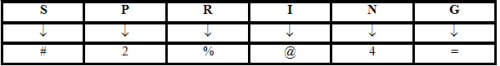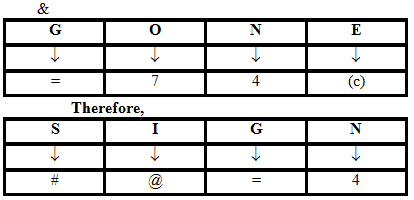TYPE – 3: CODING BASED ON RENAMING
In this type of questions, certain words are assigned different names and you are required to ascertain the code for a particular word.

Example 1: If ‘green’ is called ‘white’, ‘white’ is called ‘yellow’, ‘yellow’ is called ‘blue’, ‘blue’ is called ‘pink’ and ‘pink’ is called ‘black’, then what is the colour of milk?
(1) Green
(2) Blue
(3) Pink
(4) Yellow
Sol. (4)
The colour of milk is white. But the code of white is yellow so the answer is yellow.

Example 2: If ‘cloud’ is called ‘white’, white is called ‘rain’, rain is called ‘green’, green is called ‘air’, air is called ‘blue’, blue is called ‘water’. Where will birds fly?
(1) Air
(2) cloud
(3) White
(4) blue
Sol. (4) Birds fly in air but here air is coded as blue so the answer is blue.

TYPE – 4: CODING BASED ON GROUP OF WORDS
In this type of questions a group of words or a sentence as a whole is coded and on the basis of common words you are required to ascertain the code for each word.

Example 1: In a certain code: ‘ing ping sing’  means ‘how are you’ , ‘sing ring ding’ means ‘what about you’ and ‘ding fing ching’ means ‘what to do’ ,then how is ‘do’ written in this code language?
(1) ing
(2) fing
(3) ching
Sol: (4)
In the first and second sentence, ‘you’ is common while in the coded statement, the common word is ‘sing’. It means that sing is the code for you. Similarly, in the second and third sentence, the common word is ‘what’ and ‘ding’ respectively. From it, we can conclude that the coding for ‘what’ is ‘ding’. But for the ‘do’ we cannot assign the code because it can be coded either as’ fing’ or ‘ching’. Our answer to the question here should be ‘data inadequate’ or option number (4). Code for do is ‘fing’ or ‘ching’

Example 2: In certain code “cha pa ta “ means “I like orange” , “ga  fa pa” means “they like mango” and “fa cha ka” means “orange mango sour”, then which word stands for “Sour” ?
(1) ta
(2) ka
(3) ra
Sol. (2)
We note that the word ‘sour’ appears in the third statement and it is not repeated in any other statement. But the other words in the same statement are orange and mango which are repeated in the first and the second statement. From our observations, we note that the codes ‘cha’ and ‘fa’ are already repeated, so the only code left out which has not been assigned is ‘ka’. So code for ‘sour’ is ‘ka’.

TYPE – 5: MISCELLANEOUS
Example 1: Study the following information carefully and answer the questions given below:
The digits from 0 to 9 are coded as shown below with the exceptions that follow: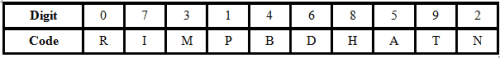Exceptions:
1. If number begins and ends with an odd digit (non-zero), then both the first and last digits are to be coded as \$.

2. If a number begins and ends with an even digit (including zero), then both the first and last digits are to be coded as #.

(1) What will be the code for 314926?
(1) MPBDHA
(2) MPBTND
(3) MPBTNA
(4) \$PBTN\$
Sol.  (2)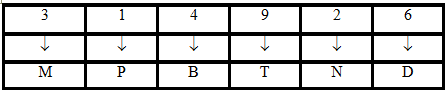(2) ‘RATHIM’ represents which of the following numbers?
(1) 095873
(2) 059673
(3) 059871
(4) 059873

Sol. (4)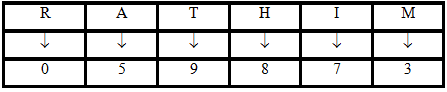(3) What will be the code for 4521736?
(1) BANPIMD
(2) BANPIRD
(3) #ANPIM#
(4) #BAMPI#

Sol.  (3)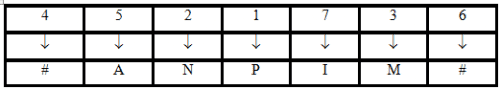Example 2: The following questions are based on substitution of letters according to the instructions of letters and conditions given below: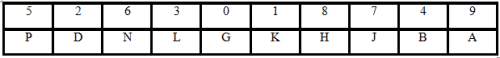However,
(i) If the number starts with non-zero odd digits, then odd digit is coded as \$.
(ii) If the number ends with 0, it is coded as @.
(iii) If the number starts with an even digit, that even digit is coded as &.

(1) What will be the code for 3840291?
(1) \$HBGDA\$
(2) \$HBGDAK
(3) LHBGDA\$
(4) SHB@DAK

Sol. (2)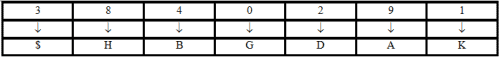(2) ‘\$PKJHB’ is the code for which of the following numbers?
(1) 251784
(2) 551784
(3) 051784

Sol. (2)  \$ is the code for any odd digit 1, 3, 5, 7 or 9
There is only one such option which starts with an odd digit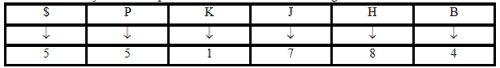(3)   What will be the code for 6374082?
(1) &LJBGHD
(2) &JHB@HD
(3) &JBBGH&
(4) &LJB@H\$

Sol. (1)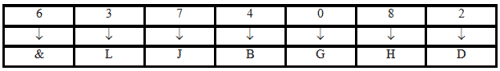(4) What will be the code for 4391760?
(1) &LAKJNG
(2) & LAKHN@
(3) &LAKHNG
(4) &LAKJN@
Sol. (4)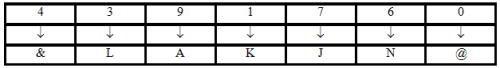Offer running on EduRev: Apply code STAYHOME200 to get INR 200 off on our premium plan EduRev Infinity!

## General Intelligence and Reasoning for SSC CHSL

10 videos|16 docs|27 tests

,

,

,

,

,

,

,

,

,

,

,

,

,

,

,

,

,

,

,

,

,

;